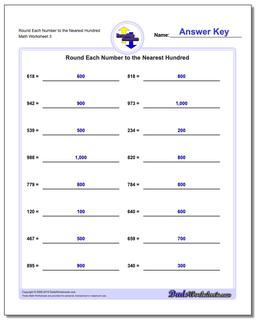# Math Worksheets: Rounding Numbers: Rounding Numbers: Round Each Number to the Nearest Hundred (Third Worksheet)## Round Each Number to the Nearest Hundred (Third Worksheet)

PropertyValue
DescriptionRound Each Number to the Nearest Hundred: Rounding worksheets where whole numbers must be rounded to the same place value as the most significant digit. This is a great starting place for building rounding concepts. (Third Worksheet)
Resource TypeWorksheet